# long division of polynomials

long division of polynomials

Emily recently learned about polynomials and now she is wondering if she can divide those polynomials in the same way as she divides two whole numbers or fractions.Do you want to help Emily with the long division of polynomials?

Let's find out how to divide polynomials through the long division method.

In this mini-lesson, we will explore the division of polynomials by learning about the long division of polynomials calculator, methods to divide using long division with the help of interesting simulation, some solved examples, and a few interactive questions for you to test your understanding.

## Lesson Plan

 1 What Do You Mean by Long Division of Polynomials? 2 Important Notes 3 Solved Examples 4 Challenging Questions 5 Interactive Questions

## What Do You Mean by Long Division of Polynomials?

In algebra, the division of algebraic expressions can be done in three ways:

• Division of a monomial by another monomial.
• Division of a polynomial by a monomial.
• Division of a polynomial by another polynomial.

A long division polynomial is an algorithm for dividing polynomial by another polynomial of the same or a lower degree.
The long division of polynomials also consists of the divisor, quotient, dividend, and the remainder as in the long division method of numbers.

Observe the numerator and denominator in the long division of polynomials as shown in the figure.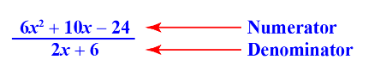The long division of polynomials also consists of a divisor, a quotient, a dividend, and a remainder.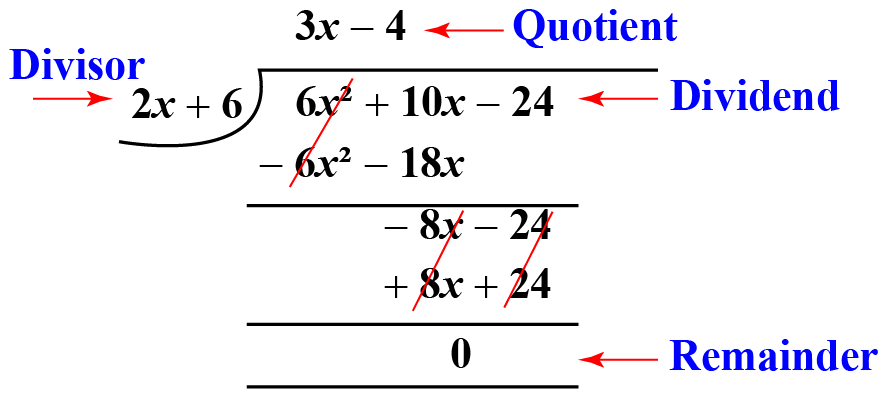## What Are the Steps For Long Division of Polynomials?

The following are the steps for the long division of polynomials:

Step 1. Arrange the terms in the decreasing order of their indices (if required). Write the missing terms with zero as their coefficient.

Step 2. For the first term of the quotient, divide the first term of the dividend by the first term of the divisor.

Step 3. Multiply this term of the quotient by the divisor to get the product.

Step 4. Subtract this product from the dividend, and bring down the next term (if any). The difference and the brought down term will form the new dividend.

Step 5. Follow this process until you get a remainder, which can be zero or of a lower index than the divisor.

Read on to see how the above steps are shown in an example.

## How to Do Long Division of Polynomials?

Let's understand how to do the long division of polynomials with an example.

We need to divide the polynomial $$a\left(x\right)={6x^4} +{3x}-{9x^2} +6$$ by the quadratic polynomial $$b\left(x\right)={x^2} - 2$$

• Arrange the polynomial in the descending order of the power of the variable.

$$a\left(x\right):{6x^4}-{9x^2} +{3x} +6$$

$$b\left(x\right):{x^2} - 2$$

•  Divide  $$a\left(x\right)$$ by $$b\left(x \right)$$ in the same way as we divide numbers.• Add the missing indices with zero (0) as the coefficient.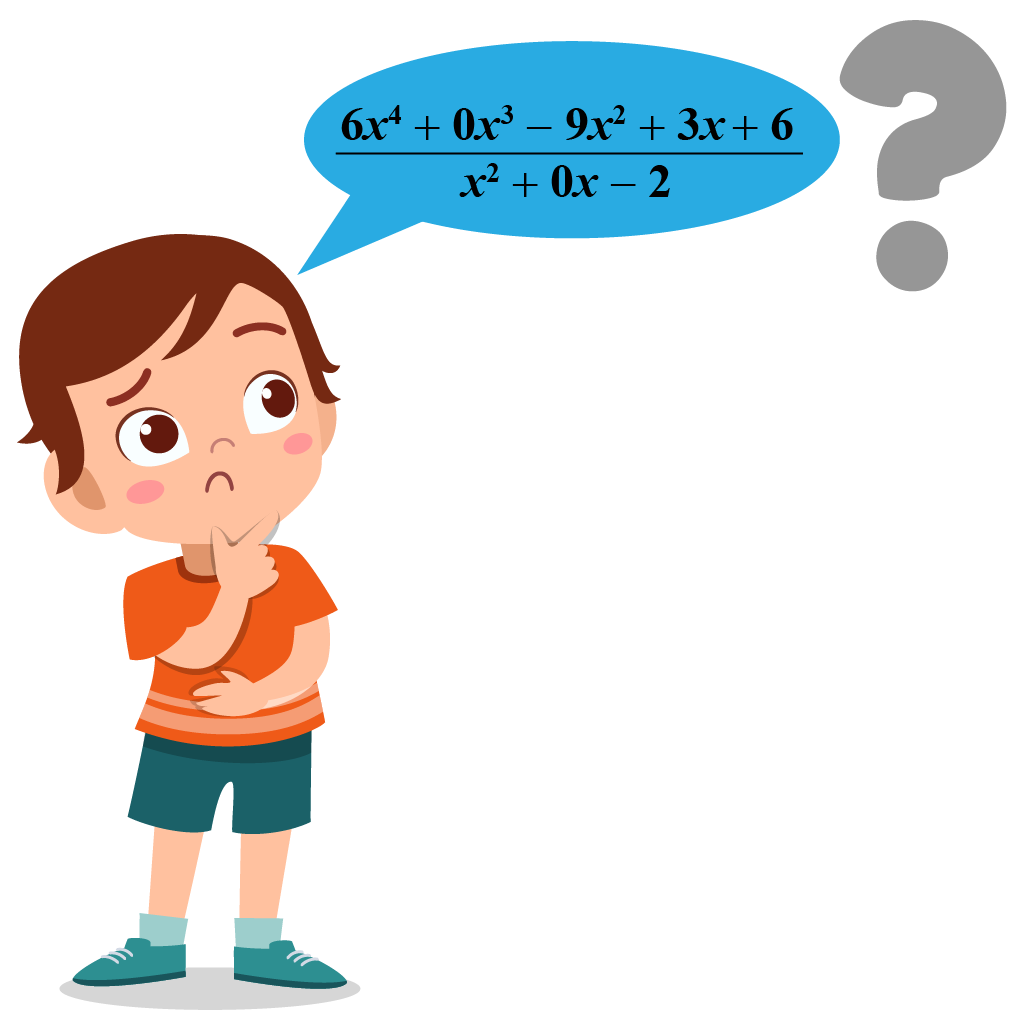• Divide $$6x^4$$ by $$x^2$$ to get the first term of the quotient.

We get $$6x^2$$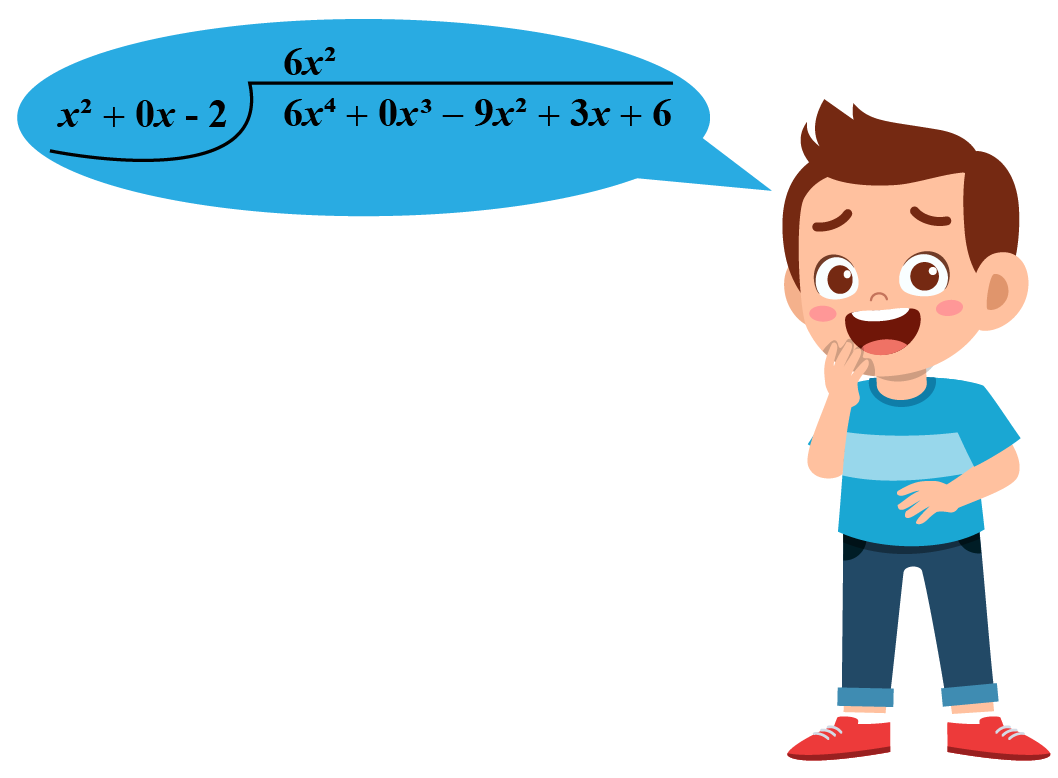• Multiply the divisor by $$6x^2$$.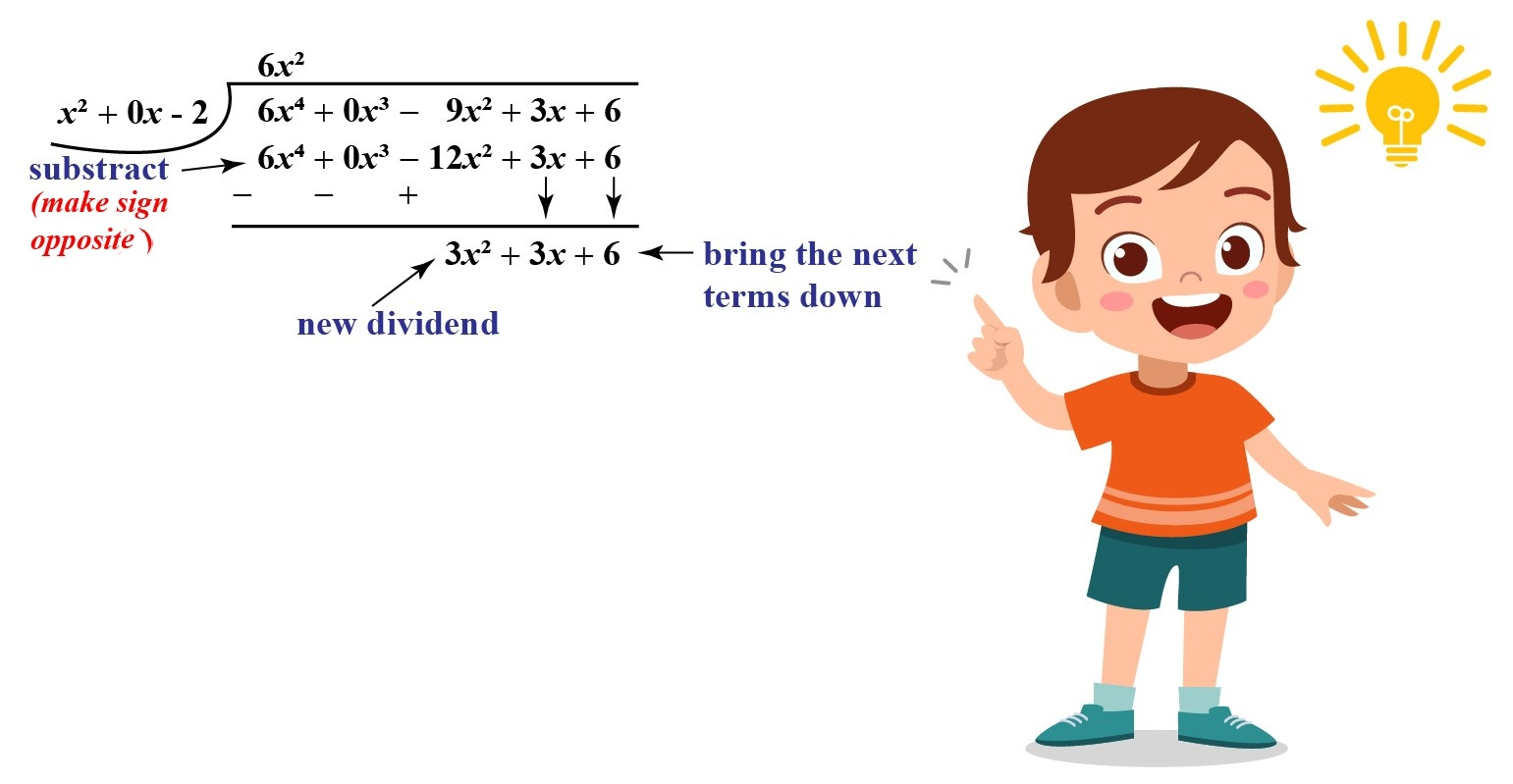• Divide $$3x^2$$ by $$x^2$$ to get the next term of the quotient.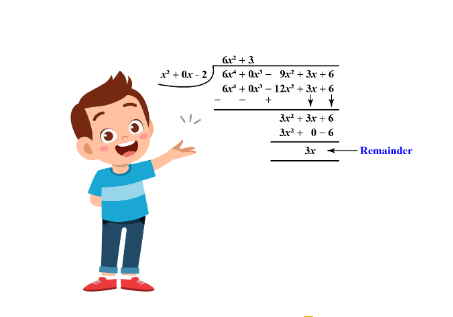• As the power of the next dividend is less than the divisor, we get our required remainder.

Note: As the remainder we got is a non-zero term, we can say that $$x^2 - 2$$ is not a factor of $${{6x^4}-{9x^2} +{3x} +6}$$

 $$\therefore$$ $$\text{The quotient is } {6x^2+3} \text{ and the remainder is } 3x$$
 Example 1

Rose wants to divide the polynomial $$(4x^3 - 3x^2 + 4x)$$ by $$2x+1$$. Can you help her with the solution?

Solution

Here, the polynomial $$4x^3 - 3x^2 + 4x$$ is divided by $$2x+1$$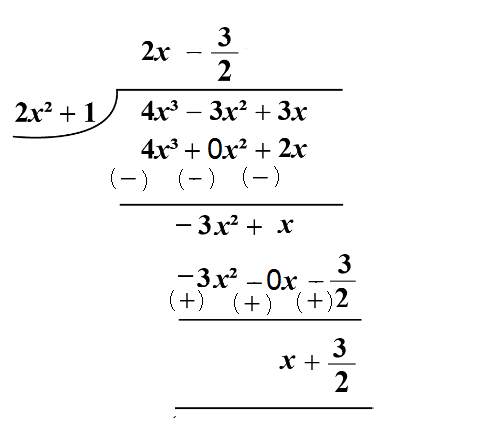$$\therefore$$ $$\text{Quotient = } 2x- \dfrac{3}{2} \text{ and remainder = } x+ \dfrac32$$
 Example 2

Solve $$(24a^2 + 48a+2) ÷ (6a + 12)$$ by using the method of long division of polynomials.

Solution

The long division of $$(24a^2 + 48a+2) ÷ (6a + 12)$$ can be done in the following way.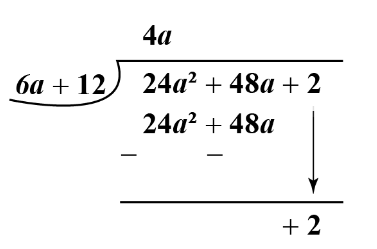$$\therefore$$ Quotient = 4a and  remainder = 2
 Example 3

Consider the following two polynomials:

\begin{align}&a\left( x \right):{x^3} - {x^2} + x - 1\\&b\left( x \right):2x + 1\end{align}

Find the quotient polynomial and the remainder when $$a\left( x \right)$$ is divided by $$b\left( x \right)$$.

Solution

We proceed as earlier:

$2x + 1\mathop{\left){\vphantom{1\begin{array}{l}{x^3} - {x^2} + x - 1\\\underline {{x^3} + \frac{1}{2}{x^2}\,\,\,\,\,\,\,\,\,\,\,\,\,\,\,\,\,\,\,\,\,} \\\,\,\,\,\,\,\,\,\,\,\,\, - \frac{3}{2}{x^2} + x - 1\\\,\,\,\,\,\,\,\,\,\,\,\, \underline {\, - \frac{3}{2}{x^2} - \frac{3}{4}x\,\,\,\,\,\,\,\,\,\,}\\\,\,\,\,\,\,\,\,\,\,\,\,\,\,\,\,\,\,\,\,\,\,\,\,\,\,\,\,\,\,\,\,\frac{7}{4}x -1\\\,\,\,\,\,\,\,\,\,\,\,\,\,\,\,\,\,\,\,\,\,\,\,\underline {\,\;\;\;\;\;\frac{7}{4}x + \frac{7}{8}\,\,\,}\\\,\,\,\,\,\,\,\,\,\,\,\,\,\,\,\,\,\,\,\,\,\,\,\,\,\,\;\;\;\;\;\;\;\;\;\;-\frac{{15}}{8}\\ \,\,\,\,\,\,\,\end{array}}}\right.\!\!\!\!\overline{\,\,\,\vphantom 1{\begin{array}{l}{x^3} - {x^2} + x - 1\\\underline {{x^3} + \frac{1}{2}{x^2}\,\,\,\,\,\,\,\,\,\,\,\,\,\,\,\,\,\,\,\,\,} \\\,\,\,\,\,\,\,\,\,\,\,\, - \frac{3}{2}{x^2} + x - 1\\\,\,\,\,\,\,\,\,\,\,\,\,\underline {\, - \frac{3}{2}{x^2}-\frac{3}{4}x\,\,\,\,\,\,\,\,\,\,} \\\,\,\,\,\,\,\,\,\,\,\,\,\,\,\,\,\,\,\,\,\,\,\,\,\,\,\,\,\,\,\,\,\frac{7}{4}x - 1\\\,\,\,\,\,\,\,\,\,\,\,\,\,\,\,\,\,\,\,\,\,\,\,\underline {\,\;\;\;\;\;\frac{7}{4}x + \frac{7}{8}\,\,\,} \\\,\,\,\,\,\,\,\,\,\,\,\,\,\,\,\,\,\,\,\,\,\,\,\,\,\,\;\;\;\;\;\;\;\;\;\; - \frac{{15}}{8}\\ & & \,\,\,\,\,\,\,\end{array}}}}\limits^{\displaystyle\,\,\, {\,\frac{1}{2}{x^2}} - {\frac{3}{4}x} +{\frac{7}{8}}\,\,\,\,\,\,\,\,\,\,\,\,\,\,}$

Thus, we have

 $$\therefore$$\begin{align}&q\left( x \right)=\frac{1}{2}{x^2} - \frac{3}{4}x + \frac{7}{8}\\&r = - \frac{{15}}{8}\end{align}Challenging Questions
•  Divide $$6x^2+10x-24$$ by $$2x+6$$ using synthetic division of polynomials.

• Divide $$60y^4 + 22y^3 − 164y^2 − 24y + 84$$ by $$6y^2 + 4y− 8$$ and find the quotient and remainder of the expression.

## Interactive Questions

Here are a few activities for you to practice. Select/Type your answer and click the "Check Answer" button to see the result.

## Let's Summarize

Mini-lesson targeted the fascinating concept of long division of polynomials. The math journey around the money starts with what a student already knows, and goes on to creatively crafting a fresh concept in the young minds. Done in a way that not only it is relatable and easy to grasp, but also will stay with them forever. Here lies the magic with Cuemath.

At Cuemath, our team of math experts is dedicated to making learning fun for our favorite readers, the students!

Through an interactive and engaging learning-teaching-learning approach, the teachers explore all angles of a topic.

Be it worksheets, online classes, doubt sessions, or any other form of relation, it’s the logical thinking and smart learning approach that we, at Cuemath, believe in.

## 1. What is the meaning of long division of polynomials?

Long division of polynomials is a technique followed in Algebra to divide a polynomial by another polynomial of a lower or the same degree.

## 2. How do you divide polynomials by long division?

Two polynomials are divided by the long division method by adapting the same technique which is used for simple division.

## 3. What is the importance of long division of polynomials?

Long division of polynomials is a way to test whether one polynomial has the other one as a factor. It also helps in breaking the dividend into a simple sequence by easy steps.

## 4. What are the advantages of long division of polynomials?

The advantage of long division of polynomials is that it is a simple and widely used method to divide two polynomials in less space and requires lesser calculations.

## 5. What are the disadvantages of long division of polynomials?

The only disadvantage of long division of polynomials is that in case the divisor is nonlinear, the calculations become more complex.

## 6.What are the main uses of a synthetic division of polynomials?

The main use of synthetic division of polynomials is that it is used when the divisor is linear and the coefficient of the variable in it is one.

More Important Topics
Numbers
Algebra
Geometry
Measurement
Money
Data
Trigonometry
Calculus